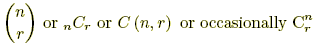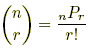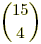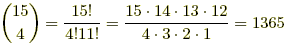# combination formula

Combination Formula

A formula for the number of possible combinations of r objects from a set of n objects. This is written in any of the ways shown below.All forms are read aloud "n choose r."

 Formula:Note:, where nPr is the formula for permutations of n objects taken r at a time. Example: How many different committees of 4 students can be chosen from a group of 15? Answer: There arepossible combinations of 4 students from a set of 15.There are 1365 different committees.